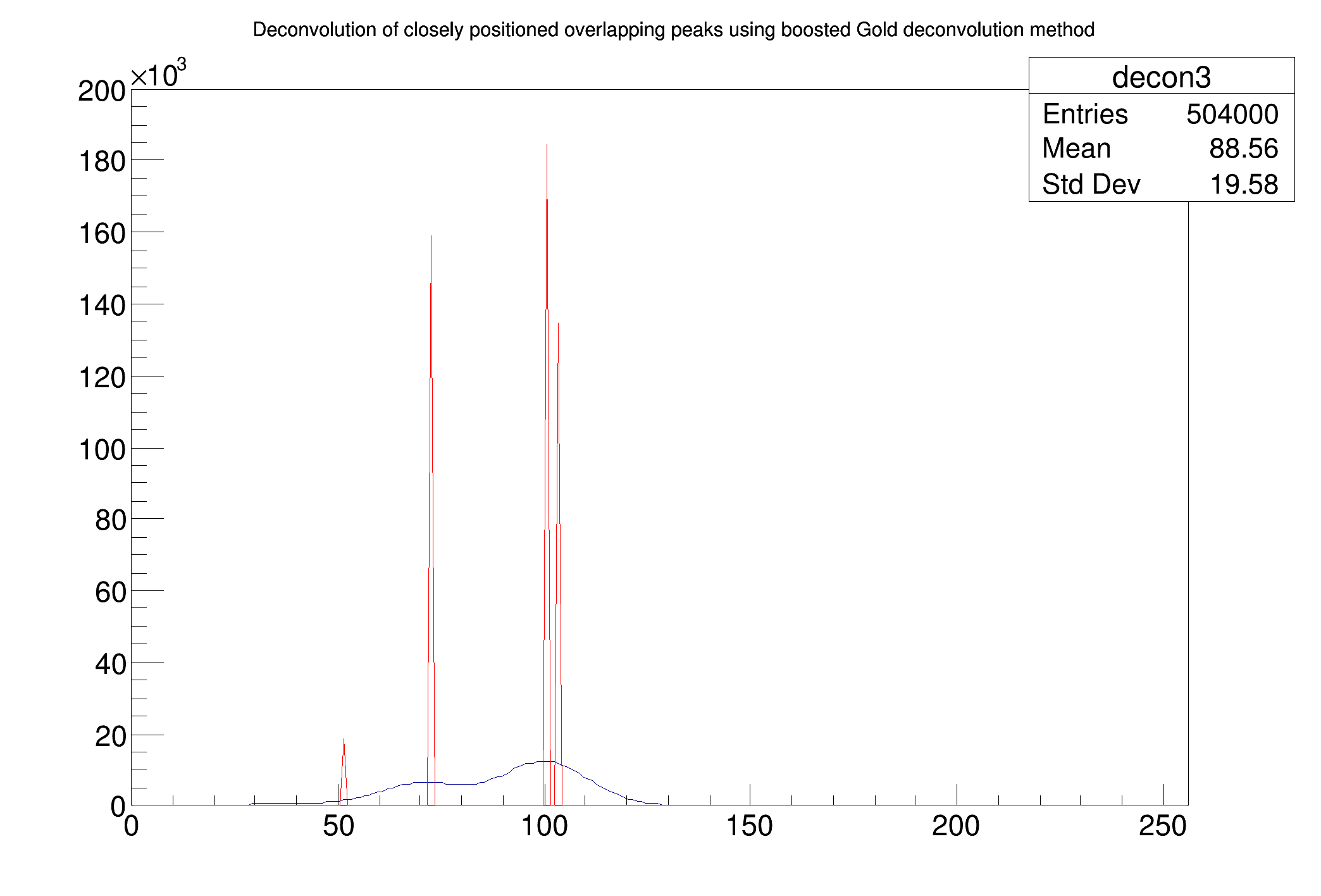ROOT   Reference Guide
Searching...
No Matches
Deconvolution_wide_boost.C File Reference

## Detailed DescriptionExample to illustrate deconvolution function (class TSpectrum).void Deconvolution_wide_boost() {
Int_t i;
const Int_t nbins = 256;
Double_t xmax = nbins;
Double_t source[nbins];
Double_t response[nbins];
gROOT->ForceStyle();
TH1F *h = new TH1F("h","Deconvolution",nbins,xmin,xmax);
TH1F *d = new TH1F("d","",nbins,xmin,xmax);
TString dir = gROOT->GetTutorialDir();
TString file = dir+"/spectrum/TSpectrum.root";
TFile *f = new TFile(file.Data());
h = (TH1F*) f->Get("decon3");
h->SetTitle("Deconvolution of closely positioned overlapping peaks using boosted Gold deconvolution method");
d = (TH1F*) f->Get("decon_response_wide");
for (i = 0; i < nbins; i++) source[i]=h->GetBinContent(i + 1);
for (i = 0; i < nbins; i++) response[i]=d->GetBinContent(i + 1);
h->SetMaximum(200000);
h->Draw("L");
TSpectrum *s = new TSpectrum();
s->Deconvolution(source,response,256,200,50,1.2);
for (i = 0; i < nbins; i++) d->SetBinContent(i + 1,source[i]);
d->SetLineColor(kRed);
d->Draw("SAME L");
}
#define d(i)
Definition RSha256.hxx:102
#define f(i)
Definition RSha256.hxx:104
#define h(i)
Definition RSha256.hxx:106
int Int_t
Definition RtypesCore.h:45
double Double_t
Definition RtypesCore.h:59
@ kRed
Definition Rtypes.h:66
float xmin
float xmax
#define gROOT
Definition TROOT.h:406
A ROOT file is a suite of consecutive data records (TKey instances) with a well defined format.
Definition TFile.h:54
1-D histogram with a float per channel (see TH1 documentation)}
Definition TH1.h:575